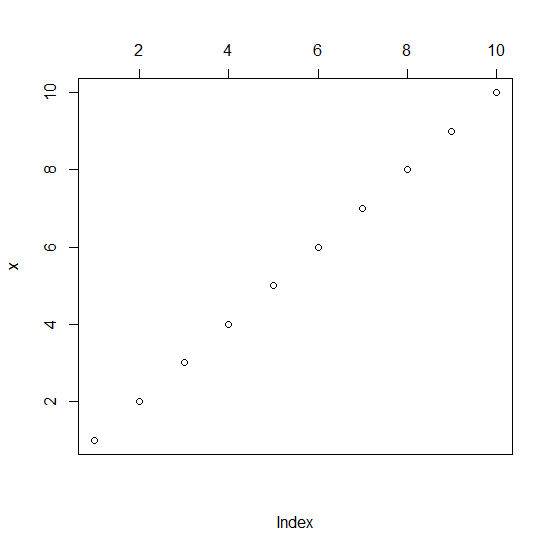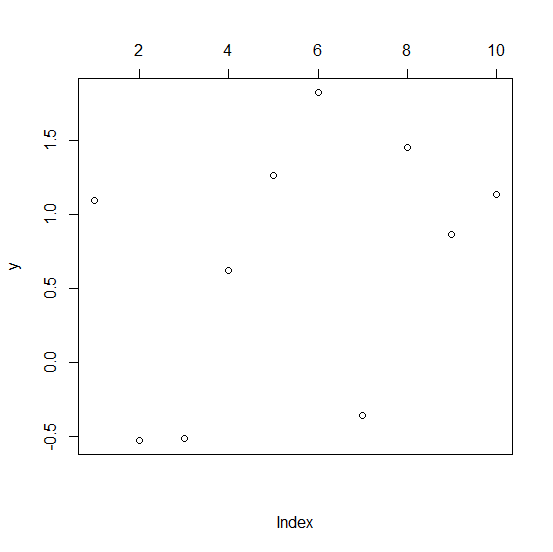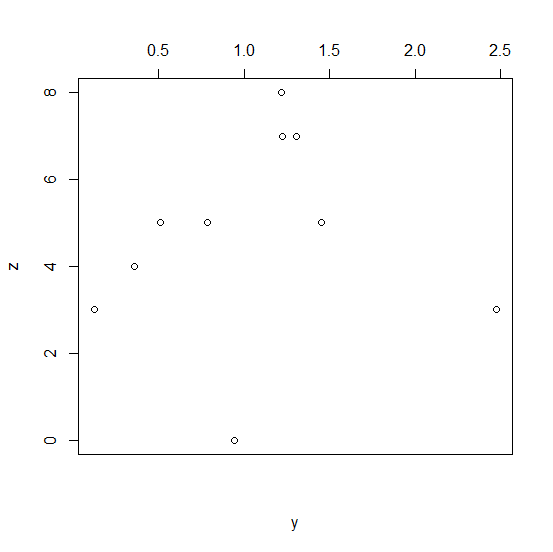# How to plot the X-axis labels on the upper-side of the plot in R?

By default, the X−axis labels are plotted on the bottom−axis of the plot and that is referred to as the first axis of axis 1, the second axis is the axis on the left−side, the third axis is the axis on the upper side of the plot, and the fourth axis is on the right−side of the plot. If we want to plot the X−axis labels on the upper−side of the plot then we can use xaxt="n" inside the plot function then define axis for upper−side using axis(3) with semi−colon.

## Example1

x<−1:10
plot(x,xaxt="n");axis(3)

## Output## Example2

y<−rnorm(10,1,0.8)
plot(y,xaxt="n");axis(3)

## Output## Example3

y<−rnorm(10,1,0.8)
z<−rpois(10,5)
plot(y,z,xaxt="n");axis(3)

## Output